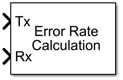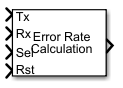# Error Rate Calculation

Compute bit error rate or symbol error rate of input data

• Library:
• Communications Toolbox / Comm Sinks

Communications Toolbox HDL Support / Comm Sinks

•## Description

The Error Rate Calculation block compares input data from a transmitter with input data from a receiver. The block calculates the error rate as a running statistic by dividing the total number of unequal pairs of data elements by the total number of input data elements from one source.

You can use this block to compute the symbol or bit error rate because it does not consider the magnitude of the difference between input data elements. If the inputs are bits, then the block computes the bit error rate. If the inputs are symbols, then the block computes the symbol error rate.

This figure shows the block with all ports enabled.## Ports

### Input

expand all

Transmitted data, specified as a scalar or column vector.

Note

If you specify the `Tx` or `Rx` input as a scalar, the object compares this value with all elements of the other input. If you specify both inputs as vectors, they must have the same size and data type.

Data Types: `single` | `double` | `int8` | `int16` | `int32` | `uint8` | `uint16` | `uint32` | `Boolean`

Received data, specified as a scalar or column vector.

Data Types: `single` | `double` | `int8` | `int16` | `int32` | `uint8` | `uint16` | `uint32` | `Boolean`

Indices of the samples to consider when comparing data, specified as a positive integer or column vector of positive integers.

#### Dependencies

To enable this input, set the Computation mode parameter to ```Select samples from input port```.

Data Types: `double`

Reset error count, specified as a scalar.

#### Dependencies

To enable this input, set the Reset port parameter to `on`.

Data Types: `double` | `Boolean`

### Output

expand all

Difference between transmitted and received data, returned as a column vector of the form ```[R; N; S]```, where:

• R is the error rate.

• N is the number of errors.

• S is the number of samples compared.

#### Dependencies

To enable this port, set the Output data parameter to `Port`.

Data Types: `double`

## Parameters

expand all

Number of samples by which the received data lags behind the transmitted data, specified as a nonnegative integer. Use this parameter to align the samples for comparison in the transmitted and received input data vectors.

Data Types: `double`

Number of data samples that the object ignores at the beginning of the comparison, specified as a nonnegative integer. Use this property to ignore the transient behavior of both input signals.

Data Types: `double`

Samples to consider, specified as one of these values.

• `Entire frame` — Compare all the samples of the received data to those of the transmitted frame.

• `Select samples from mask` — Set the indices of the samples to consider when making comparisons in the Selected samples from frame parameter.

• `Select samples from port` — Set the indices of the samples to consider when making comparisons in the `Sel` input port.

Indices of the samples to consider when comparing data, specified as a positive integer or column vector of positive integers. The default value, an empty vector, specifies that the block uses all samples from the received frame.

#### Dependencies

To enable this parameter, set the Computation mode property to ```Select samples from mask```.

Data Types: `double`

Output data location, specified as one of these options.

• `Workspace` — Send the output data to the workspace variable defined by the Variable name parameter.

• `Port` — Add an output data port to the block and send the output data to that port.

Output data variable name in the MATLAB® workspace.

#### Dependencies

To enable this parameter, set the Output data variable to `Workspace`.

Data Types: `char` | `string`

Enable the `Rst` input port.

Option to stop the simulation after the block detects the number of errors specified in the Target number of errors parameter or performs the number of comparisons specified in the Maximum number of symbols parameter.

Option to stop the simulation after detecting this number of errors, specified as a positive integer.

#### Dependencies

To enable this parameter, set the Stop simulation parameter to `on`.

Data Types: `double`

Option to stop the simulation after comparing this number of symbols, specified as a positive integer.

Note

If you use the Simulink® Coder™ rapid simulation (RSim) target to build an RSim executable, then you can tune the Target number of errors and Maximum number of symbols parameters without recompiling the model. This is useful for Monte Carlo simulations in which you run the simulation multiple times (perhaps on multiple computers) with different amounts of noise.

#### Dependencies

To enable this parameter, set the Stop simulation parameter to `on`.

Data Types: `double`

## Block Characteristics

 Data Types `Boolean` | `double` | `fixed point` | `integer` | `single` Multidimensional Signals `no` Variable-Size Signals `yes`

## Version History

Introduced before R2006a